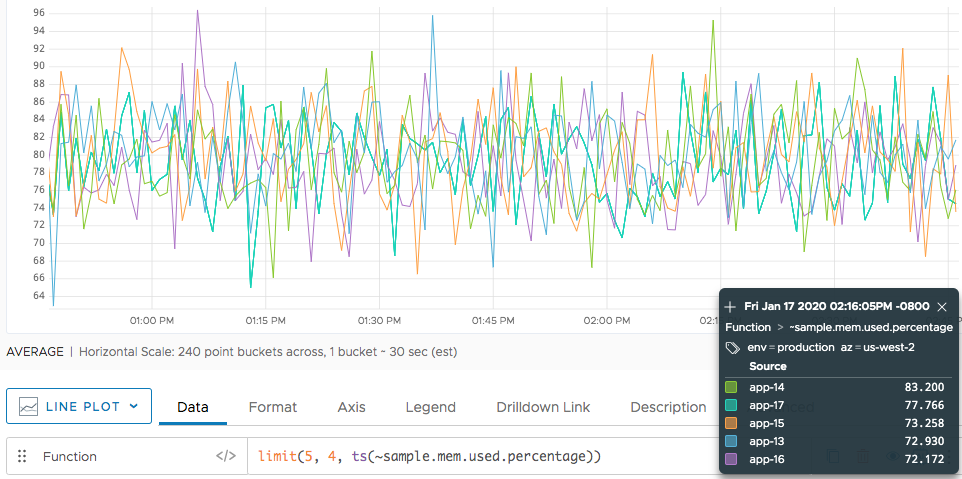Reference to the limit() function

## Summary

``````limit(<numberOfTimeSeries>[, <offsetNumber>], <tsExpression>)

limit(<numberOfHistogramSeries>[, <offsetNumber>], <hsExpression>)

limit(<numberOfTraces>, <tracesExpression>)
``````

The `limit()` function shows only a specified number of items. You can use `limit()` with time series, histograms, or traces.

 Time series filtering function Limits the number of time series displayed for `tsExpression` in a time-series chart. Histogram series filtering function Limits the number of histogram distributions displayed for `hsExpression` in a time-series chart. Traces filtering function Limits the number of traces listed by `tracesExpression` in the Traces browser.

## Parameters

### Time-Series Filtering Function

ParameterDescription
numberOfTimeSeries Number of time series that you want displayed. You can express this parameter as a number (e.g. 10) or a percentage (e.g. 17%).
tsExpression Expression that that describes the time series that you want to filter.

### Histogram Filtering Function

ParameterDescription
numberOfHistogramSeries Number of histogram series that you want displayed. You can express this parameter as a number (e.g. 10) or a percentage (e.g. 17%).
hsExpression Expression that that describes the histogram that you want to filter.

### Traces Filtering Function

ParameterDescription
numberOfTraces Number of traces that you want listed. Express this parameter as a number (e.g. 10). Do not specify a percentage.
tracesExpression Expression that that describes the traces that you want to filter. Includes a traces() function.

## Description

You can use `limit()` as a filtering function with time series, series of histogram distributions, or traces.

### Time-Series Filtering Function

The `limit()` function lets you use the `numberOfTimeSeries` parameter to specify the maximum number of time series that are returned. For example, the following query returns at most 10 time series:

`limit(10, ts(~sample.mem.used.percentage))`

Optionally, use `offsetNumber` to specify the starting index. For example, set `offsetNumber` to 5 to start with the 5th item.

### Histogram Series Filtering Function

The `limit()` function lets you use the `numberOfHistogramSeries` parameter to specify the maximum number of histogram series that are returned. For example, the following query returns at most 10 histograms:

`limit(10, ts(~sample.mem.used.percentage))`

Optionally, use `offsetNumber` to specify the starting index. For example, set `offsetNumber` to 5 to start with the 5th item.

### Traces Filtering Function

The `limit()` function lets you use the `numberOfTraces` parameter to specify the maximum number of traces that are returned. For example, the following query returns at most 50 traces:

`limit(50, traces("beachshirts.styling.makeShirts"))`

## Examples

### Time-Series Filtering Function

The following example returns only 5 of the time series.This example offsets the selection from the first example by 4. That means 1 time series is shared (the series for `app-16`), the others are different.